Courses

# SSC CPO Mock Test (GI & Reas) 3

## 50 Questions MCQ Test SSC CPO & Constable - Mock Tests & Previous Year Papers | SSC CPO Mock Test (GI & Reas) 3

Description
This mock test of SSC CPO Mock Test (GI & Reas) 3 for SSC helps you for every SSC entrance exam. This contains 50 Multiple Choice Questions for SSC SSC CPO Mock Test (GI & Reas) 3 (mcq) to study with solutions a complete question bank. The solved questions answers in this SSC CPO Mock Test (GI & Reas) 3 quiz give you a good mix of easy questions and tough questions. SSC students definitely take this SSC CPO Mock Test (GI & Reas) 3 exercise for a better result in the exam. You can find other SSC CPO Mock Test (GI & Reas) 3 extra questions, long questions & short questions for SSC on EduRev as well by searching above.
QUESTION: 1

Solution:
QUESTION: 2

Solution:
QUESTION: 3

### Find out which word or name will be in the middle after the words or names are arranged in the alphabetical order.

Solution:
QUESTION: 4
Complete the analogous pair.
Foresight : Anticipation : : Insomnia : ?
Solution:
QUESTION: 5
Choose the numeral pair which is different from others .
Solution:
QUESTION: 6
Choose the numeral pair which is different from others .
Solution:
QUESTION: 7
Complete the analogous pair.
Shoe : Shining : : Pant : ?
Solution:
QUESTION: 8
Complete the analogous pair.
Coconut : Shell : : Letter : ?
Solution:
QUESTION: 9
Choose the word which is least like the other words in the group .
Solution:
QUESTION: 10
Complete the analogous pair.
Planet : Sun :: Moon : ?
Solution:
QUESTION: 11
Four girls A, B, C and D are sitting in a circle. B and C are facing each other. Which of the following is definitely true?
Solution:
QUESTION: 12
Ashok started walking towards north. After walking 30 metres, he turned left and walked 40 metres. He then turned left and walked 30 metres. He again turned left and walked 50 metres. How far was he from his original position ?
Solution:
QUESTION: 13
The letters skipped in between the adjacent letters in the series are followed by equal space. Which of the following series observes this rule?
Solution:
QUESTION: 14

Find the number of triangles and squares in the given figure.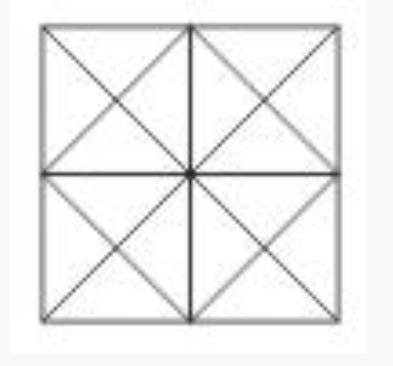Solution:

44 triangles, 10 squares

QUESTION: 15
Find the missing character.
Solution:
QUESTION: 16
Group the following figures into three classes on the basis of identical properties.
Solution: 1,7,8 are all undivided geometrical figures.
3,5,6 are geometrical figures divided into two parts.
2,4,9 are geometrical figures divided into four parts.
QUESTION: 17

Choose the odd one out.

Solution:

There are two patterns
Pattern 1
All the consecutive pairs
YZ, PQ, EF, MN, JK
Pattern 2
Second alphabet is 2 alphabets away from the last
Hence, NMK is the odd one out

QUESTION: 18
Find the missing character.
Solution:
QUESTION: 19
Find the missing character.
Solution: In fig. (A), $\left(3�3\right)+\left(6�5\right)=39.$
In fig. (B), $\left(4�4\right)+\left(5�7\right)=51.$
$\therefore$In fig. (C), missing number = $\left(3�4\right)+\left(5�5\right)=37.$
QUESTION: 20

Choose the odd one out.

Solution:
QUESTION: 21

How many such pairs of letters are there in the word CREDIBILITY each of which has only one letter between them in the word as also in the alphabet?

Solution:
QUESTION: 22
Which of the following combination of figures best represents Young creatures, Colts and Horses?
Solution:
QUESTION: 23
If 12% people in a village, are suffering from cancer and 13% from blood pressure, which of the following diagram best represents the sick population of the village?
Solution:
QUESTION: 24

In a certain code, TERMINAL is written as SDQLJOBM. How is CREDIBLE written in that code?

Solution:
QUESTION: 25

Select the correct set of symbols which will fit in the given equation 5 0 3 5 = 20.

Solution:

5 - 0 + 3 × 5
= 5 + 15 = 20

QUESTION: 26

select the correct combination of mathematical signs to replace * sign to balance the given equation.
18*3*1*26*28

Solution:
QUESTION: 27

select the correct combination of mathematical signs to replace * sign to balance the given equation.
24*3*2*12*3

Solution:
QUESTION: 28
Choose the number which is least like the other numbers in the group.
Solution:
QUESTION: 29
Choose the odd one out.
Solution:
QUESTION: 30
Choose the odd one out.
Solution:
QUESTION: 31
In this letter series, some of the letters are missing. Choose the correct letter given below -
_cb _ ca _ bacb _ ca _ bac _ d
Solution:
QUESTION: 32

Find the wrong number in the series.
16, 4, 2, 1.5, 1.75, 1.875,

Solution:

The series is ×0.25,×0.5,×0.75,×1,.......

QUESTION: 33
Choose the missing term out of the given alternatives.
AB, BA, ABC, CBA, ABCD, ?
Solution:
QUESTION: 34
In each question, three sequences of letters/numerals are given which correspond to each other. Find out the letters/numerals that come in vacant places.
C B _ _ D _ B A B C C B
_ _ 1 2 4 3 _ _ ? ? ? ?
a _ a b _ c _ b _ _ _ _
Solution:
QUESTION: 35
In the following questions some of the letters are missing. The missing letters are given in the proper sequence as one of the alternatives given under each question. Find the correct choice.
-c-ca-ab-bc-
Solution:
QUESTION: 36
In each question, three sequences of letters/numerals are given which correspond to each other. Find out the letters/numerals that come in vacant places marked by (?).
$\begin{array}{ccccccccccc}\text{_}& A& C& \text{_}& B& D& \text{_}& C& D& C& D\\ 2& \text{_}& 4& 1& \text{_}& 1& 4& \text{_}& \text{_}& \text{_}& \text{_}\\ c& d& \text{_}& b& c& \text{_}& a& ?& ?& ?& ?\end{array}$
Solution: Clearly, 4 corresponds to C and a corresponds to 4. So, a corresponds to C.
1 corresponds to D and b corresponds to 1. So, b corresponds to D.
thus, CDCD corresponds to a, b, a, b.
QUESTION: 37
In each question a statement followed by two assumptions numbered I and II is given. Consider the statement and decide which of the given assumption is implicit.
Statement : The two countries have signed a fragile pact, but the vital sovereignity issue remains unresolved.
Assumptions : I. The two countries cannot have permanent peace pact.
II. The two countries may become hostile again after a short spell of time.
Solution:
QUESTION: 38
Below is given statement followed by three conclusions numbered I, II and III. You have to consider the statement and the following conclusions and decide which of the conclusions is follows in the statement :
Statements :a. No rabbit is lion.
b. Some horses are lions.
c. All rabbits are tables.
Conclusions:I. Some tables are lions.
II. Some horses are rabbits.
III. No lion is table.
Solution:
QUESTION: 39
If Leader is coded as Actor, Actor as an Administrator, Administrator as a Traitor, Traitor as a Social-reformer, then who takes part in the Parliamentary elections?
Solution:
QUESTION: 40
If the 25th of August in a year in Thursday, the number of Mondays in that month is
Solution: 25th August is a Thursday. So, 22nd August is a Monday. So, Mondays fall on 1st, 8th, 15th, 22nd and 29th of August. Thus, there are five Mondays.
QUESTION: 41
In each question, five words are given. Four of them are alike in some way. One is different from them.Which is the one that does not belong to that group?
Solution:
QUESTION: 42
Choose one word which cannot be made from the letters of the given word
REPRIMAND
Solution:
QUESTION: 43

In the following questions, select a figure from amongst the four alternatives, which when placed in the blank space of fig. (X) would complete the pattern.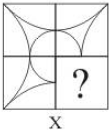Solution:
QUESTION: 44

In each question below is given a group of letters followed by four combinations of digits marked (A), (B), (C) and (D). You have to find out which of the combinations correctly represents the group of letters based on the following coding system and mark the letter of that combination as the answer. If none of the four combinations correctly represents the group of letters, mark (E) i.e. 'None of these' as the answer.
Letter : C F H L M A G J D K
Digits :  2  6  4  8  0  7  3  9  1  5

DFMKHLG

Solution:

G→3
C→2
A→7
J→9
H→4
M→0
K→5

QUESTION: 45

In each question below is given a group of letters followed by four combinations of digits marked (A), (B), (C) and (D). You have to find out which of the combinations correctly represents the group of letters based on the following coding system and mark the letter of that combination as the answer. If none of the four combinations correctly represents the group of letters, mark (E) i.e. 'None of these' as the answer.
Letter : C F H L M A G J D K
Digits :  2  6  4  8  0  7  3  9  1  5

GCAJHMK

Solution:

G→3
C→2
A→7
J→9
H→4
M→0
K→5

QUESTION: 46

Complete the analogous pair.

31. 4 : 27 :: 9 : ?

Solution:

22 : 33 : : 32 : ?
So the answer should be 43 = 64

QUESTION: 47

Select the correct mirror image of the figure (X) from amongst the given alternatives.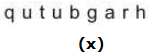Solution:
QUESTION: 48

Find out from amongst the four alternatives as to how the pattern would appear when the transparent sheet is folded at the dotted line.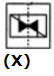Solution:
QUESTION: 49

Each of the following question consist of five problem figure marked 1, 2, 3, 4, 5 followed by Answer figure A, B, C, D and E. Select of figure from the answer figure which will continue the same series as given in the problem figure.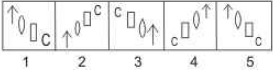Solution:
QUESTION: 50

In the questions, figure (X) is embedded in one of following figures. Find out the correct alternative.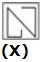Solution: